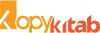# CALCULUS 2 [BSc Mathematics Paper 2 – Golden Series by Nirali]

140.00CALCULUS 2 [BSc Mathematics Paper 2 – Golden Series by Nirali]

140.00

 Authors Name Dr. Shrikisan Gaikwad , Dr. Kalyanrao Takale , Dr. Pravin Jadhav , Dr, Vikas Jadhav , Prof. Veena P. Kshirsagar , Prof. S. R. Patil , Prof. S. M. Waingade ISBN 13 9789389686593 Publisher Nirali Prakashan Edition First Buy E-Book (PDF) [Click on Logo >>]Pages 134 Language English Publishing Year Dec-19
For E-Books purchased from Kopykitab.com For E-Books purchased from Amazon
##### Description

Unit 1: Differentiation,
Unit 2: L’Hospital Rule and Successive Differentiation,
Unit 3: Ordinary Differential Equations,
Unit 4: Exact Differential Equations

This book is based on a course Calculus-II. The purpose of this text book is to provide a rigorous treatment of the foundations of differential calculus. We write this book as per the revised syllabus of F.Y. B.Sc. Mathematics, revised by Savitribai Phule Pune University, Pune, implemented from June 2019. Calculus is the most useful subject in all of mathematics and it is used extensively in applied mathematics and engineering.

In Chapter 1, we develop the theory of differentiation of real valued function of single variable. Fur- thermore, we study algebraic properties of derivative. We prove Interior extremum theorem, Mean Value theorems and their Consequences, Intervals of increasing and decreasing of a function, rst derivative test for extrema and as an applications of Mean Value theorems we solved some examples. The Chapter 2, is devoted for Indeterminate forms, L’ Hospital Rules, Taylor theorem and Maclaurin theorem with Lagranges form of remainder. Also, we study Successive Differentiation and obtain the nth derivatives of functions. We proved Leibnitz theorem for successive differentiation and solve some examples.